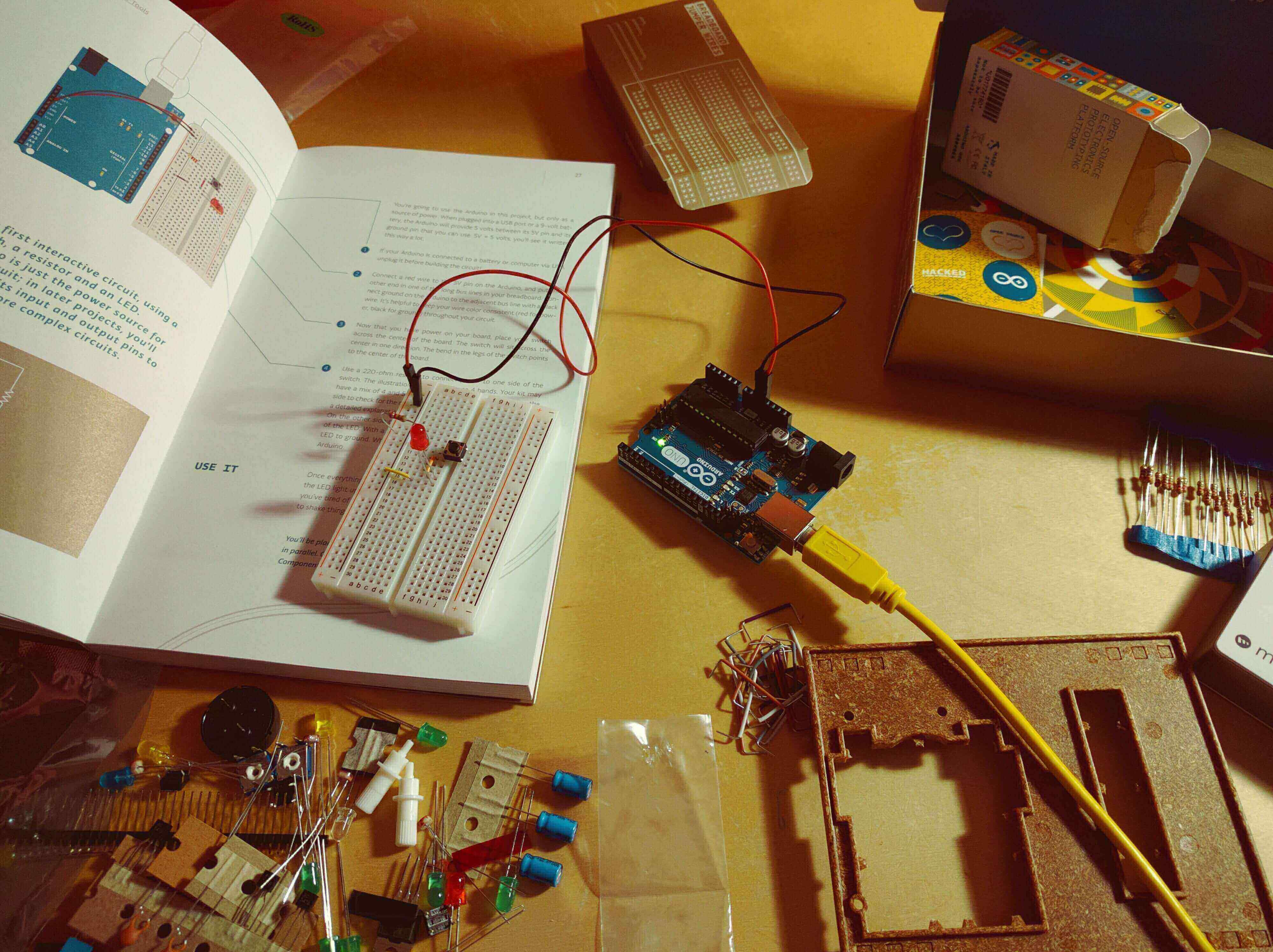# Control System ( EC-404 )

( EC- 4th Sem )### Course Outcomes

After Completion of this Course students can:

• Understand concepts of Control System.
• Understand concepts of Feedback characteristics of control systems.
• Understand concepts of Time response analysis.
• Understand concepts of Time domain stability analysis.
• Understand concepts of Frequency response analysis.
• Understand concepts of Frequency domain stability analysis.
• Be able to solve System Design Questions.
• Understand Concepts of State space representation of systems.

### Course Content

• Terminology and classification of control system, examples of control system, mathematical modeling of mechanical and electrical systems, differential equations, transfer function, block diagram representation and reduction, signal flow graph techniques.
Feedback characteristics of control systems Open loop and closed loop systems, effect of feedback on control system and on external disturbances, linearization effect of feedback, regenerative feedback.
• Standard test signals, time response of 1st order system, time response of 2nd order system, steady-state errors and error constants, effects of additions of poles and zeros to open loop and closed loop system.
Time domain stability analysis Concept of stability of linear systems, effects of location of poles on stability, necessary conditions for stability, Routh-Hurwitz stability criteria, relative stability analysis, Root Locus concept, guidelines for sketching Root-Locus.
• s Correlation between time and frequency response, Polar plots, Bode Plots, all-pass and minimum-phase systems, log-magnitude versus Phase-Plots, closed-loop frequency response.
Frequency domain stability analysis : Nyquist stability criterion, assessment of relative stability using Nyquist plot and Bode plot (phase margin, gain margin and stability).
• Approaches to system design Design problem, types of compensation techniques, design of phase-lag, phase lead and phase lead-lag compensators in time and frequency domain, proportional, derivative, integral and Composite Controllers.
• State space representation of systems, block diagram for state equation, transfer function decomposition, solution of state equation, transfer matrix, relationship between state equation and transfer function, controllability and observability.

### Book References

• Albert D. Helfrick, William David Cooper, “Modern electronic instrumentation and measurement techniques”, TMH 2008.
• Oliver Cage, “Electronic Measurements and Instrumentation”, TMH, 2009.
• Alan S. Morris, “Measurement and Instrumentation Principles”, Elsevier (Buterworth Heinmann), 2008.
• David A. Bell, “Electronic Instrumentation and Measurements”, 2nd Ed., PHI, New Delhi 2008.
• H.S. Kalsi, “Electronics Instrumentation”, TMH Ed. 2004.
• A.K.Sawhney, “A Course in Electrical and Electronic Measurements and Instrumentation”, Dhanpat Rai.
• MMS Anand, “Electronic Instruments & Instrumentation Technology”, PHI Pvt. Ltd., New Delhi Ed. 2005.

### List of Experiments

• Control System performance analysis and applications of MATLAB in Control system performance analysis & design.

• Exp -1
• Exp -2
• Exp -3
• Exp -4
• Exp -5
• Exp -6
• Exp -7
• Exp -8
• Exp -9
• Exp -10

### Cousre Notes

For "Course Notes" you can refer our Notes Section for 2nd Year( Click Here )

• TAGS:

•College Academy
Author- Himanshu
( Core Member )